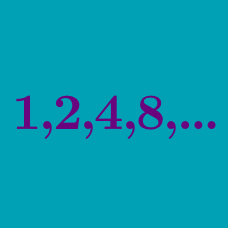Algebra

# Geometric Progressions Warmup

If $\{a_n\}$ is a geometric progression with $a_1 = 5$ and $a_5 = 80$, what is the value of $a_3$?

If $a_1, 3, a_3, 12, a_5$ is a geometric progression, which of the following is a possible value of $a_5?$

The geometric mean of the geometric sequence ${2, a_2, a_3}$ is 10. What is the value of $a_3?$

Note. The geometric mean of three numbers $a_1, a_2, a_3$ is $\sqrt{a_1\cdot a_2 \cdot a_3}.$ For example, the geometric mean of $2, 4,$ and $8$ is $\sqrt{2\cdot(4)\cdot8} = \sqrt{64} = 4.$

• $x, y, z^2$ is a geometric progression.

• Each of $x, y, z$ are integers.

• $x + y + z^2 < 120$.

• Find the largest possible value of $x + y + z^2$.

If $a_1, 5, a_3, 45, a_5$ is a geometric progression, which of the following is a possible value of $a_3?$

×